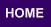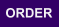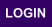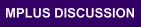Mplus
Sunday
October 01, 2023Mplus Mplus at a GlanceGeneral DescriptionMplus Programs Pricing Version History System Requirements Platforms
 Analyses/ResearchMplus Examples Papers References
 Search

Mplus Web Notes

1. Muthén, B. (2002). Using Mplus Monte Carlo simulations in practice: A note on assessing estimation quality and power in latent variable models. Version 2, March 22, 2002.
2. Muthén, B. and Asparouhov, T. (2002). Using Mplus Monte Carlo simulations in practice: A note on non-normal missing data in latent variable models. Version 2, March 22, 2002.
3. Muthén, B. and Asparouhov, T. (2002). Modeling of Heteroscedastic Measurement Errors. Version 1, July 19, 2002.
4. Muthén, B. and Asparouhov, T. (2002). Latent Variable Analysis With Categorical Outcomes: Multiple-Group And Growth Modeling In Mplus. Version 5, December 9, 2002.
5. Muthén, B. (2002). Supplementary Analyses for the Article "Assessment of Treatment Effects Using Latent Variable Modeling: Comments on the New York School Choice Study". Version 1, December 20, 2002.
6. Muthén, B. and Asparouhov, T. (2003). Modeling interactions between latent and observed continuous variables using maximum-likelihood estimation in Mplus. Version 1, March 5, 2003.
7. Asparouhov, T. (2004). Weighting for unequal probability of selection in latent variable modeling. Mplus Web Notes: No. 7. Refer to Mplus Papers for the abstract. Mplus inputs and outputs used in section 10 of this paper can be downloaded from here. MLwiN runs used in section 10 of this paper can be downloaded from here. HLM runs used in section 10 of this paper can be downloaded from here.
8. Asparouhov, T. (2004). Weighting for unequal probability of selection in multilevel modeling. Mplus Web Notes: No. 8. Refer to Mplus Papers for the abstract.
9. Asparouhov, T. (2004). Stratification in multivariate modeling. Mplus Web Notes: No. 9. December 16, 2004. Refer to Mplus Papers for the abstract. Mplus inputs and outputs used in this paper can be downloaded from here.
10. Asparouhov, T. & Muthén, B. (2006). Robust Chi Square Difference Testing with mean and variance adjusted test statistics. Mplus Web Notes: No. 10. May 26, 2006.
11. Asparouhov, T. & Muthén, B. (2006). Constructing covariates in multilevel regression. Mplus Web Notes: No. 11. February 15, 2006.
12. Asparouhov, T. & Muthén, B. (2010). Computing the strictly positive Satorra-Bentler chi-square test in Mplus. Paper can be downloaded from here. Mplus Web Notes: No. 12. January 24, 2012.
13. Muthén, B. & Asparouhov, T. (2011). LTA in Mplus: Transition probabilities influenced by covariates. Mplus Web Notes: No. 13. July 27, 2011.
14. Asparouhov, T. & Muthén, B. (2012). Using Mplus TECH11 and TECH14 to test the number of latent classes. Mplus Web Notes: No. 14. May 22, 2012.
15. Asparouhov, T. & Muthén, B. (2014). Auxiliary variables in mixture modeling: Three-step approaches using Mplus. Structural Equation Modeling: A Multidisciplinary Journal, 21:3, 329-341. DOI: 10.1080/10705511.2014.915181. The posted version corrects several typos in the published version. An earlier version of this paper was posted as web note 15. Appendices with Mplus scripts are available here.
16. Asparouhov, T. & Muthén, B. (2012). Multiple group multilevel analysis. Mplus Web Notes: No. 16. November 15, 2012.
17. Muthén, B. & Asparouhov, T. (2013). BSEM measurement invariance analysis. Mplus Web Notes: No. 17. January 11, 2013.
18. Asparouhov, T. & Muthén, B. (2014). Multiple-group factor analysis alignment. Structural Equation Modeling: A Multidisciplinary Journal, 21:4, 495-508. DOI: 10.1080/10705511.2014.919210. An earlier version of this paper was posted as web note 18. Mplus input, output, and data files for Web Note 18 Monte Carlo simulations are available here. Real-data analyses of the tradition-conformity items for 26 countries and corresponding Monte Carlo simulation are available here. For further information, see the 2013 July Ljubljana talk here.
19. Asparouhov, T. & Muthén B. (2015). Structural equation models and mixture models with continuous non-normal skewed distributions. Structural equation models and mixture models with continuous non-normal skewed distributions. Structural Equation Modeling: A Multidisciplinary Journal, DOI: 10.1080/10705511.2014.947375. An earlier version of this paper was posted as web note 19. Mplus inputs and outputs used in this paper can be downloaded here.
20. Asparouhov, T. & Muthén, B. (2014). Using Mplus individual residual plots for diagnostic and model evaluation in SEM. Mplus Web Notes: No. 20. October 6, 2014. Revised October 31, 2017.
21. Asparouhov, T. & Muthén, B. (2020). Auxiliary variables in mixture modeling: Using the BCH method in Mplus to estimate a distal outcome model and an arbitrary secondary model. Mplus Web Notes: No. 21. May 14, 2014. Revised February 4, 2021.
22. Asparouhov, T, & Muthén, B. (2017). Prior-Posterior Predictive P-values. Mplus Web Notes: No. 22. Version 2. April 27, 2017. (Download Mplus analyses)
23. Asparouhov, T, & Muthén, B. (2019). Latent variable interactions using maximum-likelihood and Bayesian estimation for single- and two-level models. Mplus Web Notes: No. 23. May 3, 2019. (Download the Section 4 Bayes examples).

Type of Analysis Input file Data file View output
1. Muthén, B. (2002). Using Mplus Monte Carlo simulations in practice: A note on assessing estimation quality and power in latent variable models. Version 2, March 22, 2002. Contact the author. Download this web note or the corresponding set of examples.
Study A. Linear growth model: no covariates, no missing data mca.inp N/A mca.std
Study B. Linear growth model: covariate, no missing data mcb.inp N/A mcb.std
Study C. Linear growth model: covariate, MAR missing data mcc.inp N/A mcc.std
Back to the top

Type of Analysis Input file Data file View output
2. Muthén, B. and Asparouhov, T. (2002). Using Mplus Monte Carlo simulations in practice: A note on non-normal missing data in latent variable models. Version 2, March 22, 2002. Contact the first author. Download this web note or the corresponding set of examples.
Study A. Linear growth model with non-normal data: no covariate, no missing data, ML estimator mc2a.inp N/A mc2a.std
Study A. Linear growth model with non-normal data: no covariate, no missing data, MLR estimator mc2b.inp N/A mc2b.std
Study B. Linear growth model with non-normal data: covariate, MAR missing data, ML estimator mc2c.inp N/A mc2c.std
Study B. Linear growth model with non-normal data: covariate, MAR missing data, MLR estimator mc2d.inp N/A mc2d.std
Back to the top

Type of Analysis Input file Data file View output
3. Muthén, B. and Asparouhov, T. (2002). Modeling of Heteroscedastic Measurement Errors. Version 1, July 19, 2002. Contact the first author. Download this web note or the corresponding set of examples.
Model 1. Full model. 5 parameters. model1.inp stationary_oddsratio.dat model1.std
Back to the top

Type of Analysis Input file Data file View output
4. Muthén, B. and Asparouhov, T. (2002). Latent Variable Analysis With Categorical Outcomes: Multiple-Group And Growth Modeling In Mplus. Version 5, December 9, 2002. Contact the first author. Download this web note or the corresponding set of examples.
Example 1. ASB EFA catex1.inp asb.dat catex1.std
Example 2. ASB CFA catex2.inp asb.dat catex2.std
Example 3. ASB factor analysis (MIMIC) with gender as covariate catex3.inp asb.dat catex3.std
Example 4. ASB factor analysis (MIMIC) with gender as covariate and gender non-invariance catex4.inp asb.dat catex4.std
Example 5. ASB multiple-group analysis catex5.inp asb.dat catex5.std
Example 6. ASB multiple-group analysis with gender non-invariance catex6.inp asb.dat catex6.std
Study A. Multiple-group modeling: full invariance catstudya.inp mc.dat catstudya.std
Study B. Multiple-group modeling: partial threshold invariance catstudyb.inp mc.dat catstudyb.std
Study C. Growth modeling: binary outcomes catstudyc.inp mcgrowth.dat catstudyc.std
Study D. Growth modeling: polytomous outcomes catstudyd.inp mcgrowth.dat catstudyd.std
Study E. Growth modeling: multiple-indicator, polytomous outcomes catstudye.inp mcgrowth3.dat catstudye.std
Back to the top

Type of Analysis Input file Data file View output
5. Muthén, B. (2002). Supplementary Analyses for the Article "Assessment of Treatment Effects Using Latent Variable Modeling: Comments on the New York School Choice Study". Version 1, December 20, 2002. Contact the author. Download this web note or the corresponding set of examples.
Study A. 2-class scenario with 3 time points jasaa.inp N/A jasaa.std
Study B. 3-class scenario with 3 time points jasab.inp N/A jasab.std
Study C. 2-class scenario with 2 time points jasac.inp N/A jasac.std
Back to the top

Type of Analysis Input file Data file View output
6. Muthén, B. and Asparouhov, T. (2003). Modeling interactions between latent and observed continuous variables using maximum-likelihood estimation in Mplus. Version 1, March 5, 2003. Contact the first author. Download this web note or the corresponding set of examples.
Example 1. Monte Carlo example web6ex1.inp N/A web6ex1.std
Example 2. Cross-sectional example of behavior web6ex2.inp web6cross.dat web6ex2.std
Example 3. Growth modeling example web6ex3.inp web6growth.dat web6ex3.std
Back to the top

Type of Analysis Input file Data file View output
10. Asparouhov, T. and Muthen, B. (2006). Robust Chi Square Difference Testing with Mean and Variance Adjusted Test Statistics. Mplus Web Notes: No. 10. May 26, 2006. Contact the second author. Download this web note or the corresponding set of examples.

The set of examples includes a DOS batch file called ALL.BAT which executes Step 2 and Step 3 for each data set created in Step 1. The DOS batch file uses a utility called Extractor to extract the p-value for the Chi-Square Test for Difference Testing from the H0 analysis in Step 3. The p-values are extracted and placed into the file pval.txt. The data sets created in Step 1 are not included.

Step 1: Data generation gendata.inp N/A gendata.out
Step 2: H1 analysis (shown for one data set) difinp1.inp mcdiff.dat difinp1.out
Step 3: H0 analysis (shown for one data set) difinp0.inp mcdiff.dat difinp0.out
Back to the top

Type of Analysis Input file Data file View output
12. Asparouhov, T. and Muthen, B. (2010). Computing the strictly positive Satorra-Bentler chi-square test in Mplus. Contact the second author. Download this web note.
Example 1, M0 model run1.inp 1.dat run1.out
Example 1, M1 model run2.inp 1.dat run2.out
Example 1, M10 model run3.inp 1.dat run3.out
Example 2, M0 model run4.inp 1.dat run4.out
Example 2, M1 model run5.inp 1.dat run5.out
Example 2, M10 model run6.inp 1.dat run6.out
Example 3, M0 model run7.inp 2.dat run7.out
Example 3, M1 model run8.inp 2.dat run8.out
Example 3, M10 model run9.inp 2.dat run9.out
Example 4, M0 model run10.inp 2.dat run10.out
Example 4, M1 model run11.inp 2.dat run11.out
Example 4, M10 model run12.inp 2.dat run12.out
Back to the top